Saturday, December 4, 2021
Home > IIT JEE Main and Advance > JEE Main 2020 Question Paper Answer Keys Solutions 4 September Evening Shift

# JEE Main 2020 Question Paper Answer Keys Solutions 4 September Evening Shift

Hi Students, welcome to Amans Maths Blogs (AMB). On this post, you will get JEE Main 2020 Question Paper Answer Keys Solutions 4 September Evening Shift. As you know that Joint Entrance Examination means JEE Main and JEE Advanced is one of the most important entrance exam in engineering entrance examination. JEE Main 2020 is Computer Based Test (CBT) and it was conducted by the National Testing Agency (NTA). JEE Main 2020 exam duration is three hours and the exam consists of 300 marks (Maximum Marks). JEE Main 2020 Questions Paper has 2 sections in all three Subject Papers (Physics, Chemistry, Mathematics) and each subject has 25 questions.

## JEE Main 2020 Physics Questions Paper with Answer Keys & Solutions

In JEE Main 2020 Question Paper Answer Keys Solutions 4 September Evening Shift, Physics question paper’s answer keys and solutions by Resonance.

JEE Main 2020 Physics Question Paper with Answer Keys and Solutions (By Resonance)

## JEE Main 2020 Chemistry Questions Paper with Answer Keys & Solutions

In JEE Main 2020 Question Paper Answer Keys Solutions 4 September Evening Shift, Chemistry question paper’s answer keys and solutions by Resonance.

JEE Main 2020 Chemistry Question Paper with Answer Keys and Solutions (By Resonance)

## JEE Main 2020 Mathematics Questions Paper with Answer Keys & Solutions

In JEE Main 2020 Question Paper Answer Keys Solutions 4 September Evening Shift, Mathematics question paper’s answer keys and solutions by Amans Maths Blogs (AMB).

Instructions for SECTION 1 : (Maximum Marks : 80):

This section contains 20 multiple choice questions. Each question has 4 choices (1), (2), (3) and (4) for its answer, out of which Only One is correct.

JEE Main 2020 Mathematics Questions with Answer Keys and Solutions : Ques No 1

Let f : (0, ∞) → (0, ∞) be a differentiable function such that f(1) = e and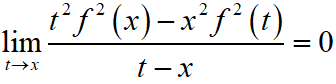. If f(x) = 1, then x is equal to :

Options:

A. 1/e

B. 2e

C. 1/2e

D. e

Solution:

JEE Main 2020 Maths Questions Answer Keys Solutions 4th Sep Shift 2 : Ques No 2

Contrapositive of the statement :

If a function f is differentiable at a, then it is also continuous at a’, is :

Options:

A. If a function f is not continuous at a, then it is not differentiable at a.

B. If a function f is continuous at a, then it is differentiable at a.

C. If a function f is not continuous at a. then it is differentiable at a.

D. If a function f is continuous at a, then it is not differentiable at a.

Solution:

JEE Main 2020 Mathematics Questions with Answer Keys and Solutions : Ques No 3

The solution of the differential equation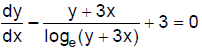is (where C is a constant of integration).

Options:

A. x – 2loge(y + 3x) = C

B. x – loge(y + 3x) = C

C. y + 3x – [(logex)2]/2 = C

D. y – [(loge(y + 3x))2 ]/2 = C

Solution:

JEE Main 2020 Maths Questions Answer Keys Solutions 4th Sep Shift 2 : Ques No 4

If for some positive integer n, the coefficients of three consecutive terms in the binomial expansion of (1 + x)n+5 are in the ratio 5 : 10 : 14, then the largest coefficient in the expansion is :

Options:

A. 330

B. 252

C. 792

D. 462

Solution:

JEE Main 2020 Mathematics Questions with Answer Keys and Solutions : Ques No 5

The circle passing through the intersection of the circles, x2 + y2 – 6x = 0 and x2 + y2 – 4y = 0, having its centre on the line, 2x – 3y + 12 = 0, also passes through the point :

Options:

A. (1, –3)

B. (–1, 3)

C. (–3, 6)

D. (–3, 1)

Solution:

JEE Main 2020 Maths Questions Answer Keys Solutions 4th Sep Shift 2 : Ques No 6

In a game two players A and B take turns in throwing a pair of fair dice starting with player A and total of scores on the two dice, in each throw is noted. A wins the game if he throws a total of 6 before B throws a total of 7 and B wins the game if he throws a total of 7 before A throws a total of six. The game stops as soon as either of the players wins. The probability of A winning the game is:

Options:

A. 30/61

B. 5/6

C. 5/31

D. 31/61

Solution:

JEE Main 2020 Mathematics Questions with Answer Keys and Solutions : Ques No 7

The angle of elevation of a cloud C from a point P, 200 m above a still take is 30°. If the angle of
depression of the image of C in the lake from the point P is 60°, then PC (in m) is equal to

Options:

A. 100

B. 400√3

C. 200√3

D. 400

Solution:

JEE Main 2020 Maths Questions Answer Keys Solutions 4th Sep Shift 2 : Ques No 8

The function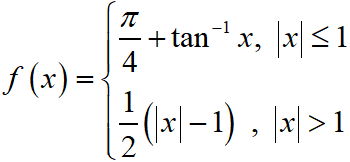is

Options:

A. continuous on R – {–1} and differentiable on R – {–1, 1}.

B. both continuous and differentiable on R – {–1}.

C. both continuous and differentiable on R – {1}

D. continuous on R – {1} and differentiable on R – {–1, 1}.

Solution:

JEE Main 2020 Mathematics Questions with Answer Keys and Solutions : Ques No 9

Suppose the vectors x1, x2 and x3 are the solutions of the system of linear equations, Ax = b when the vector b on the right side is equal to b1, b2 and b3 respectively. If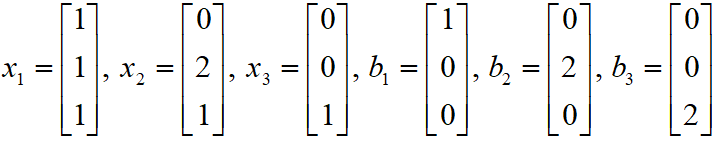then the determinant of A is equal of A is equal to :

Options:

A. 3/2

B. 4

C. 1/2

D. 2

Solution:

JEE Main 2020 Maths Questions Answer Keys Solutions 4th Sep Shift 2 : Ques No 10

Let a1, a2, …. an be a given A.P. whose common difference is an integer and Sn = a1 + a2 + … + an. If a1 = 1, an = 300 and 15 ≤ n ≤ 50, then the ordered pair (Sn–4, an–4) is equal to :

Options:

A. (2490, 248)

B. (2490, 249)

C. (2480, 249)

D. (2480, 248)

Solution:

JEE Main 2020 Mathematics Questions with Answer Keys and Solutions : Ques No 11

Let, where each Xi contains 10 elements and each Yi contains 5 elements. If each element of the set T is an element of exactly 20 of sets Xi’s and exactly 6 of sets Yi‘s then n is equal to :

Options:

A. 45

B. 15

C. 30

D. 50

Solution:

JEE Main 2020 Maths Questions Answer Keys Solutions 4th Sep Shift 2 : Ques No 12

The area (in sq. units) of the largest rectangle ABCD whose vertices A and B lie on the x-axis and vertices C and D lie on the parabola, y = x2 – 1 below the x-axis, is :

Options:

A. 1/3√3

B. 4/3

C. 4/3√3

D. 2/3√3

Solution:

JEE Main 2020 Mathematics Questions with Answer Keys and Solutions : Ques No 13

Let x = 4 be a directrix to an ellipse whose centre is at the origin and its eccentricity is 1/2. If P(1, β) β > 0 is a point on this ellipse, then the equation of the normal to it at P is

Options:

A. 7x– 4y = 1

B. 4x – 2y = 1

C. 8x – 2y = 5

D. 4x – 3y = 2

Solution:

JEE Main 2020 Maths Questions Answer Keys Solutions 4th Sep Shift 2 : Ques No 14

The distance of the point (1, –2, 3) from the plane x – y + z = 5 measured parallel to the line x/2 = y/3 = z/-6 is

Options:

A. 1/7

B. 7

C. 1

D. 7/5

Solution:

JEE Main 2020 Mathematics Questions with Answer Keys and Solutions : Ques No 15

If the perpendicular bisector of the line segment joining the points P(1, 4) and Q(k, 3) has y-intercept equal to –4, then a value of k is ;

Options:

A. √15

B. –4

C. –2

D. √14

Solution:

JEE Main 2020 Maths Questions Answer Keys Solutions 4th Sep Shift 2 : Ques No 16

The integral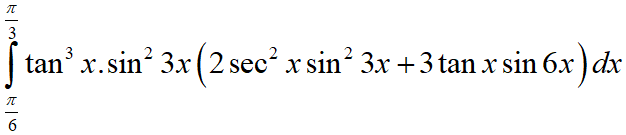is equal to

Options:

A. -1/9

B. 9/2

C. -1/18

D. 7/18

Solution:

JEE Main 2020 Mathematics Questions with Answer Keys and Solutions : Ques No 17

The minimum value of 2sinx + 2cosx is :

Options:

A.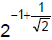B.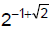C.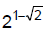D.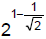Solution:

JEE Main 2020 Maths Questions Answer Keys Solutions 4th Sep Shift 2 : Ques No 18

If a and b are real numbers such that (2 + α)4 = a + bα, where α = (-1 + i√3) / 2, then (a + b) is equal to

Options:

A. 33

B. 24

C. 9

D. 57

Solution:

JEE Main 2020 Mathematics Questions with Answer Keys and Solutions : Ques No 19

Let λ ≠ 0 be in R. If α and β are the roots of the equation, x2 – x + 2λ = 0 and α and γ are the roots of the equation, 3x2 – 10x + 27λ = 0, then βγ / λ is equal to

Options:

A. 27

B. 36

C. 9

D. 18

Solution:

JEE Main 2020 Maths Questions Answer Keys Solutions 4th Sep Shift 2 : Ques No 20

If the system of equations
x + y + z = 2
2x + 4y – z = 6
3x + 2y + λz = μ,

Options:

A. λ + 2μ = 14

B. 2λ – μ = 5

C. 2λ + μ = 14

D. λ – 2μ = -5

Solution:

Instructions for SECTION 2 : (Maximum Marks : 20):

This section contains FIVE (05) questions. The answer to each question is NUMERICAL VALUE with two digit integer and decimal upto one digit.

If the numerical value has more than two decimal places truncate/round-off the value upto TWO decimal places.

Full Marks : +4 If ONLY the correct option is chosen.

Zero Marks : 0 In all other cases.

JEE Main 2020 Mathematics Questions with Answer Keys and Solutions : Ques No 21

Let PQ be a diameter of the circle x2 + y2 = 9. If α and β are the lengths of the perpendiculars from P and Q on the straight line, x + y = 2 respectively, then the maximum value of αβ is ……

Solution:

JEE Main 2020 Maths Questions Answer Keys Solutions 4th Sep Shift 2 : Ques No 22

If the variance of the following frequency distribution :

Class :         10-20          20-30          30-40
Frequency :     2                 x                 2

is 50, then x is equal to …..

Solution:

JEE Main 2020 Mathematics Questions with Answer Keys and Solutions : Ques No 23

A test consists of 6 multiple choice questions, each having 4 alternative answers of which only one is correct. The number of ways, in which a candidate answers all six questions such that exactly four of the answers are correct, is ……

Solution:

JEE Main 2020 Maths Questions Answer Keys Solutions 4th Sep Shift 2 : Ques No 22

Let {x} and [x] denote the fractional part of x and the greatest integer ≤ x respectively of a real number x.

If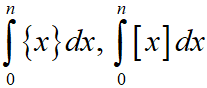and 10(n2 – n), (n ∈ N, n > 1) are three consecutive terms of a G.P. then n is equal

Solution:

JEE Main 2020 Mathematics Questions with Answer Keys and Solutions : Ques No 25

If a = 2i + j + 2k, then the value of |i x (a x i)|2 + |j x (a x j)|2 + |k x (a x k)|2 is equal to